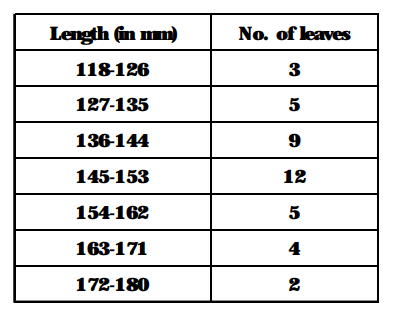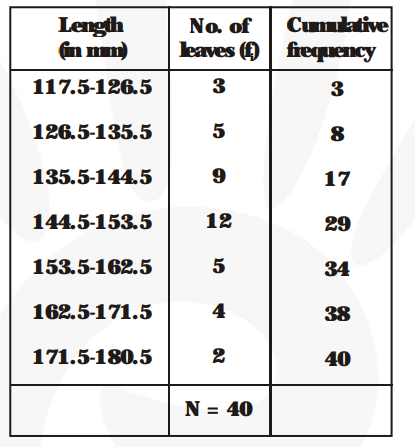# The length of 40 leaves of a plant are measured correct to the nearest millimetre,

Question:

The length of 40 leaves of a plant are measured correct to the nearest millimetre, and the data obtained is represented in the following table. Find the median length of the leaves.Solution:

The given series is in inclusive form. We may prepare the table in exclusive form and prepare the cumulative frequency table as given below :Here, N = 40

$\therefore \quad \frac{\mathbf{N}}{\mathbf{2}}=20$

The cumulative frequency just greater than 20 is 29 and the corresponding class is 144.5- 153.5.

So, the median class is 144.5-153.5.

$\therefore \ell=144.5, \mathrm{~N}=40, \mathrm{C}=17, \mathrm{f}=12$ and $\mathrm{h}=9$

$\therefore \ell=144.5, \mathrm{~N}=40, \mathrm{C}=17, \mathrm{f}=12$ and $\mathrm{h}=9$

Therefore, median $=\ell+\left\{\frac{\frac{\mathbf{N}}{\mathbf{2}}-\mathbf{C}}{\mathbf{f}}\right\} \times \mathbf{h}$

$=144.5+\frac{(\mathbf{2 0}-\mathbf{1 7})}{\mathbf{1 2}} \times 9=144.5+\frac{\mathbf{3} \times \mathbf{9}}{\mathbf{1 2}}$

= 144.5 + 2.25 = 146.75

Hence, median length of leaves is 146.75 mm